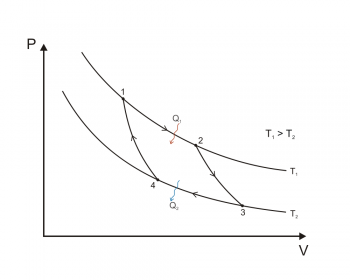Thermodynamic ProcessesA thermodynamic process is the evolution of certain properties, which are called thermodynamic properties, in relation to a particular thermodynamic system. In order to study a thermodynamic process, it is required that the system be in thermodynamic equilibrium at the initial and final point of the process; that is, that the magnitudes that undergo a variation when passing from one state to another must be completely defined in their initial and final states.

In this way thermodynamic processes can be interpreted as the result of the interaction of one system with another after being eliminated some ligature between them, so that finally the systems are in balance (mechanical, thermal and / or material) with each other.

In a less abstract way, a thermodynamic process can be seen as the changes of a system, from initial conditions to other final conditions, due to its destabilization.

A thermodynamic process can be reversible or irreversible. All real transformations are irreversible, since frictions can not be completely eliminated, so the reversibility condition is only a theoretical approximation.

System in thermodynamic equilibrium

A thermodynamic system is in principle in a state of thermodynamic equilibrium when the main variables of the system (ie pressure, volume and temperature) do not experience any additional variation over time.

In a thermodynamic process it occurs at the moment when two or all of the previous variables change. Keep in mind that the variation of only one of them is impossible because they are all interconnected by a ratio of inverse or direct proportion. This thermodynamic transformation will take the system to another point of equilibrium.

For this reason, the initial and final state of a transformation are identified by two pairs of values ​​of the three quantities that define the state of a body: pressure, volume or temperature.

Energy exchange in a thermodynamic process

In a thermodynamic process, three different situations can occur in terms of energy exchange:

• Exchanging work, but without exchanges of heat (for an adiabatic system: adiabatic transformation)
• Exchanging heat, but without exchanging work; (for example, for an Isocora transformation)
• Exchanging work and heat (for example, for an isobaric transformation or an isotherm)

Types of thermodynamic processes

For the classification of types of thermodynamic processes, it is often interesting to group the thermodynamic processes in pairs in which each variable that remains constant is a member of a conjugate pair of thermodynamic variables.

Pressure-volume

The conjugate pair pressure-volume has to do with the transfer of mechanical or dynamic energy as a result of the work.

• An isobaric process takes place at constant pressure. In other words, the system is dynamically connected, with a movable border, to a deposit at constant pressure. When a perfect gas evolves isobarically from a state A to a state B, the associated temperature and volume follow Charles's law.
• An isochoric process takes place at constant volume, so that the work done by the system will be zero. This implies that the process does not perform pressure-volume work. It follows that, by a simple two-dimensional system, any thermal energy transferred to the system externally absorbs in the form of internal energy. It can be said that the system is dynamically isolated from the environment by a rigid boundary.

Temperature-entropy

The conjugate pair temperature-entropy in a thermodynamic process has to do with the transfer of thermal energy as a result of warming.

• An isothermal process (or isothermal process) takes place at a constant temperature. In other words, the system is thermally connected, by a thermally conductive boundary, to a constant temperature reservoir.
• An adiabatic process is a process in which there is no energy added or subtracted from the system by heating or cooling. For a reversible process, this is identical to an isentropic process. It can be said that the system is thermally isolated from its environment and that its boundary is a thermal insulator. If the system has an entropy that has not yet reached its maximum equilibrium value, the entropy will increase, even if the system is thermally isolated.
• An isentropic process takes place at constant entropy. For a reversible process, this is identical to an adiabatic process. If the system has an entropy that has not yet reached the maximum equilibrium value, a cooling process may be needed to maintain this entropy value.

Chemical potential - number of particles

The processes of the sections have implicitly assumed that the borders are also impervious to particles. It can be assumed that the borders are rigid and thermally insulated, but they are permeable to one or more types of particles. By chemical potential pair - number of particles these considerations are maintained; This conjugate pair has to do with the transfer of energy through the transfer of particles.

• In a process of constant chemical potential the system is connected by transfer of particles with a permeable border to the particles.
• In a constant particle number process there is no energy added or subtracted from the system by particle transfer. It can be said that the system is isolated by transfer of particles from its surroundings through a permeable border to the particles.
valoración: 3 - votos 1

Last review: March 13, 2019